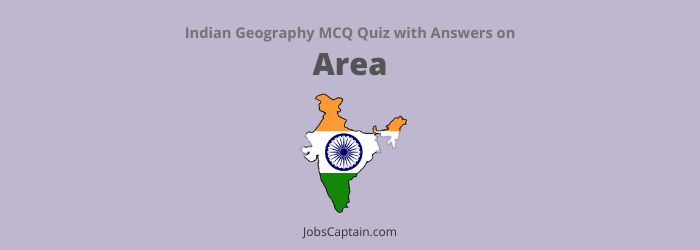# MCQ on Area: Indian Geography QuizQuestion 1. How many villages are (approximately) there in India?

(A) 5 lakh

(B) 8 lakh

(C) 6 lakh 30 thousand

(D) None of the above

(A) 5 lakh

Question 2. Area of India is 2.4% of the total area of the world but its__________.

(A) Population is 28% of world population

(B) Population is 18% of world population

(C) Population is 17% of world population

(D) Population is 16% of world population

(C) Population is 17% of world population

Question 3. Where does India rank in area among the countries of the world?

(A) Eighth

(B) Seventh

(C) Sixth

(D) Fifth

(B) Seventh

Question 4. India is in size___________.

(A) Fifth largest country of the world

(B) Seventh largest country of the world

(C) Sixth largest country of the world

(D) None of the above

(B) Seventh largest country of the world

Question 5. Which of the following pair is not correctly matched?

(A) Number of states in India – 26

(B) Longitudinal extent – 68º 7′ E – 97º 25′ E

(C) Latitudinal extent – 8º4′ N – 37º6′ N

(D) Total area of India – 3.28 million sq. km.

(A) Number of states in India – 26

Question 6. Which of the following statements are true about India?

1. India is the fifth largest country of the world.
2. It occupies about 2.4% of the total area of the lithosphere.
3. Whole of India lies in the tropic zone.
4. 82º30′ East longitude is used to determine Indian Standard Time.

Use the code given below to select the correct answer.

(A) 2 and 4

(B) 1 and 3

(C) 2 and 3

(D) 1 and 2